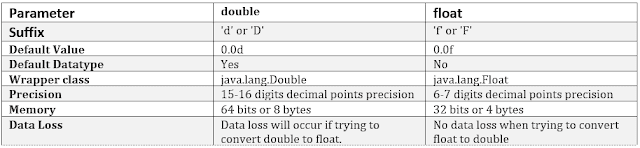-->

Difference between double and float in java 

Both double and float are used to represent floating-point numbers in java, although there are similarities as well as differences between double and float in java.

double can provide precision up to 15 to 16 decimal points whereas float provides precision up to 6 to 7 decimal places.

double is more expensive in case of storage requirements. It requires 8 bytes to store a variable whereas float takes 4 bytes to store a variable.

If memory is a constraint then it's better to use float than double.

Here is one of the best book to learn Java: Core Java Volume 1 - Fundamentals by Cay S. Horstmann to learn more about similarities and differences between a float and a double data type in Java.Let's discuss the differences in detail.

Difference between double and float in Java

1. Suffix

By default, float numbers are double in java. In order to store them in the float variable, you need to explicitly add the suffix 'f' or 'F'.

Suppose we have the number 3.123456. Let's see what happens if we store it in the float variable number.
float number = 3.123456 //compile time error
There are two options to correct the above compiler error. First by adding 'f' or 'F' at the end of the number
float number = 3.123456f
//OR
float number = 3.123456F
Second by typecasting it to float, i.e
float number = (float) 3.123456
For double you need to explicitly add the suffix 'd' or 'D'.
1.345 , 1.4d or 1.6D

2. Default Value

Default value for float in 0.0f and Default value for double is 0.0d.

3. Default Datatype

double is the default data type of floating-point literals and float is not the default data type.

4. Wrapper Class

Wrapper class for double is java.lang.Double. Wrapper class for float is java.lang.Float.

5. Precision

double is a double-precision floating-point operation that gives 15-16 decimal points precision.

float is a single-precision floating-point operation that gives 6-7 digits decimal points precision.

You can get the details about the single and double-precision floating-point operation here.

Double Precision Example

public class DoublePrecisionExample {
public static void main(String[] args) {
double number = 3.1234567891011121314;
System.out.println("double precision upto 15-16 digits after decimal : ");
System.out.print(number);
}
}

Output:

double precision upto 15-16 digits after decimal :
3.123456789101112
As we can see in the output it only prints up to 3.123456789101112 and leaving 1314.

Float Precision Example

public class FloatPrecisionExample {
public static void main(String[] args) {
float number = 3.12345678910f;
System.out.println("float precision upto 6-7 digits after decimal : ");
System.out.print(number);
}
}

Output:

float precision upto 6-7 digits after decimal :
3.1234567
As we can see in the output it only prints up to 3.1234567 and leaving 8910.

6. Memory

double takes 8 bytes(64 bits) where as float takes 4 bytes (32 bits).
Lets see through Example

Double Datatype Size

public class DoubleDatatypeSize {
public static void main(String[] args) {
System.out.println("Double Size in Bytes : "+ Double.BYTES);
System.out.println("Double Size in Bits : "+ Double.SIZE);
}
}

Output:

Double Size in Bytes : 8
Double Size in Bits : 64

Float Datatype Size

public class FloatDatatypeSize {
public static void main(String[] args) {
System.out.println("Float Size in Bytes : "+ Float.BYTES);
System.out.println("Float Size in Bits : "+ Float.SIZE);
}
}

Output:

Float Size in Bytes : 4
Float Size in Bits : 32

7. Data Loss

There will be data loss if we convert double to float as double has a wider range than float.
There will be no data loss if we do the opposite that is from float to double.

Similarities Between double and float in java

1. Both double and float data types are used to represent real numbers in java that is numbers with decimal values.
2. Both are not precise and provide approximate values.
3. We should use logical operators(> or <) to compare these values as these are not precise.

When to use what: float or double?

If we have memory concerns that it's better to use float than double.
If memory is not a concern or we require precision more than 7 then double is preferable.

Conclusion

Here is a quick overview of all the points we discussDifference between double and float in java

That's all about the difference between double and float in Java. Remember, by default floating-point numbers are double in Java, if you want to store them into float variables, you need to either cast them explicitly or suffixed them using the 'f' or 'F' character.

It's also best practice to choose a data type which takes less storage if it's sufficient for data you are storing, so choose float over double if you are happy with precision and range, double is more accurate then float though.

Thanks for reading this tutorial so far. If you like this tutorial then please share it with your friends and colleagues. If you have any questions, doubts, suggestions, or feedback then please drop a comment and I'll try to answer your question.